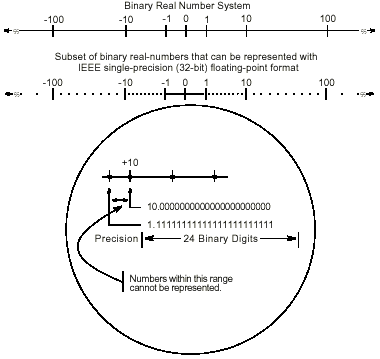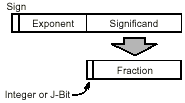# Floating Point

The following text is a replica of chapter 7.2 of the Intel Architecture Software Developer's Manual Volume 1: Basic Architecture. If you are interested in the complete manual you can download it from http://www.intel.com, Order Number 243190.

## 1. Real Numbers and Floating-Point Formats

This section describes how real numbers are represented in floating-point format in the IA FPU. It also introduces terms such as normalized numbers, denormalized numbers, biased exponents, signed zeros, and NaNs.

### 1.1 Real Number System

As shown in Figure 1, the real-number system comprises the continuum of real numbers from minus infinity (-∞) to plus infinity (+∞).Figure 1: Binary Real Number System

Because the size and number of registers that any computer can have is limited, only a subset of the real-number continuum can be used in real-number calculations. As shown at the bottom of Figure 1, the subset of real numbers that a particular FPU supports represents an approximation of the real number system. The range and precision of this real-number subset is determined by the format that the FPU uses to represent real numbers.

### 1.2 Floating-Point Format

To increase the speed and efficiency of real-number computations, computers or FPUs typically represent real numbers in a binary floating-point format. In this format, a real number has three parts: a sign, a significand, and an exponent. Figure 2 shows the binary floating-point format that the IA FPU uses. This format conforms to the IEEE standard.Figure 2: Binary Floating-Point Format

The sign is a binary value that indicates whether the number is positive (0) or negative (1). The significand has two parts: a 1-bit binary integer (also referred to as the J-bit) and a binary fraction. The J-bit is often not represented, but instead is an implied value. The exponent is a binary integer that represents the base-2 power that the significand is raised to.

Table 1 shows how the real number 178.125 (in ordinary decimal format) is stored in floating-point format. The table lists a progression of real number notations that leads to the single-real, 32-bit floating-point format (which is one of the floating-point formats that the FPU supports). In this format, the significand is normalized (refer to Section Normalized Numbers) and the exponent is biased (refer to Section Biased Exponent). For the single-real format, the biasing constant is +12710.

 Notation Value Ordinary Decimal 178.125 Scientific Decimal 1.78125 E 102 Scientific Binary 1.0110010001 E 2111 Scientific Binary (Biased Exponent) 1.0110010001 E 210000110 Single-Real Format Sign Biased Exponent Normalized Significand 0 10000110 01100100010000000000000 (1. implied => J-Bit)

Table 1. Real Number Notation

### 1.3 Normalized Numbers

In most cases, the FPU represents real numbers in normalized form. This means that except for zero, the significand is always made up of an integer of 1 and the following fraction:

1.fff...ff

For values less than 1, leading zeros are eliminated. (For each leading zero eliminated, the exponent is decremented by one.)

Representing numbers in normalized form maximizes the number of significant digits that can be accommodated in a significand of a given width. To summarize, a normalized real number consists of a normalized significand that represents a real number between 1 and 2 and an exponent that specifies the number's binary point.

### 1.4 Biased Exponent

The FPU represents exponents in a biased form. This means that a constant is added to the actual exponent so that the biased exponent is always a positive number. The value of the biasing constant depends on the number of bits available for representing exponents in the floating-point format being used. The biasing constant is chosen so that the smallest normalized number can be reciprocated without overflow. For 32-bit real numbers the bias of the exponent is +12710.

### 1.5 Real Number and Non-number Encodings

A variety of real numbers and special values can be encoded in the FPUÕs floating-point format. These numbers and values are generally divided into the following classes:

• Signed zeros.
• Denormalized finite numbers.
• Normalized finite numbers.
• Signed infinities.
• NaNs.
• Indefinite numbers.

(The term NaN stands for "Not a Number.")

Figure 3 shows how the encodings for these numbers and non-numbers fit into the real number continuum. The encodings shown here are for the IEEE single-precision (32-bit) format, where the term "S" indicates the sign bit, "E" the biased exponent, and "F" the fraction. (The exponent values are given in decimal.) The FPU can operate on and/or return any of these values, depending on the type of computation being performed. The following sections describe these number and non-number classes.Figure 3: Real Numbers and NaNs

### 1.6 Signed Zeros

Zero can be represented as a +0 or a -0 depending on the sign bit. Both encodings are equal in value. The sign of a zero result depends on the operation being performed and the rounding mode being used. Signed zeros have been provided to aid in implementing interval arithmetic. The sign of a zero may indicate the direction from which underflow occurred, or it may indicate the sign of an infinity (°) that has been reciprocated.

### 1.7 Normalized and Denormalized Finite Numbers

Non-zero, finite numbers are divided into two classes: normalized and denormalized. The normalized finite numbers comprise all the non-zero finite values that can be encoded in a normalized real number format between zero and infinity (∞). In the single-real format shown in Figure 3, this group of numbers includes all the numbers with biased exponents ranging from 1 to 25410 (unbiased, the exponent range is from -12610 to +12710 ).

When real numbers become very close to zero, the normalized-number format can no longer be used to represent the numbers. This is because the range of the exponent is not large enough to compensate for shifting the binary point to the right to eliminate leading zeros.

When the biased exponent is zero, smaller numbers can only be represented by making the integer bit (and perhaps other leading bits) of the significand zero. The numbers in this range are called denormalized (or tiny) numbers. The use of leading zeros with denormalized numbers allows smaller numbers to be represented. However, this denormalization causes a loss of precision (the number of significant bits in the fraction is reduced by the leading zeros).

When performing normalized floating-point computations, an FPU normally operates on normalized numbers and produces normalized numbers as results. Denormalized numbers represent an underflow condition.

A denormalized number is computed through a technique called gradual underflow. Table 2 gives an example of gradual underflow in the denormalization process. Here the single-real format is being used, so the minimum exponent (unbiased) is -12610. The true result in this example requires an exponent of -12910 in order to have a normalized number. Since -12910 is beyond the allowable exponent range, the result is denormalized by inserting leading zeros until the minimum exponent of -12610 is reached.

 Operation Sign Exponent* Significand True Result 0 -129 1.01011100000...00 Denormalize 0 -128 0.10101110000...00 Denormalize 0 -127 0.01010111000...00 Denormalize 0 -126 0.00101011100...00 Denormal Result 0 -126 0.00101011100...00
NOTE: * Expressed as an unbiased, decimal number.

Table 2: Denormalization Process

In the extreme case, all the significant bits are shifted out to the right by leading zeros, creating a zero result.

The FPU deals with denormal values in the following ways:

• It avoids creating denormals by normalizing numbers whenever possible.

• It provides the floating-point underflow exception to permit programmers to detect cases when denormals are created.

• It provides the floating-point denormal-operand exception to permit procedures or programs to detect when denormals are being used as source operands for computations.

When a denormal number in single- or double-real format is used as a source operand and the denormal exception is masked, the FPU automatically normalizes the number when it is converted to extended-real format.

### 1.8 Signed Infinities

The two infinities, +∞ and -∞, represent the maximum positive and negative real numbers, respectively, that can be represented in the floating-point format. Infinity is always represented by a zero significand (fraction and integer bit) and the maximum biased exponent allowed in the specified format (for example, 25510 for the single-real format).

The signs of infinities are observed, and comparisons are possible. Infinities are always inter-preted in the affine sense; that is, -∞ is less than any finite number and +∞ is greater than any finite number. Arithmetic on infinities is always exact. Exceptions are generated only when the use of an infinity as a source operand constitutes an invalid operation.

Whereas denormalized numbers represent an underflow condition, the two infinity numbers represent the result of an overflow condition. Here, the normalized result of a computation has a biased exponent greater than the largest allowable exponent for the selected result format.

### 1.9 NaNs

Since NaNs are non-numbers, they are not part of the real number line. In Figure 3, the encoding space for NaNs in the FPU floating-point formats is shown above the ends of the real number line. This space includes any value with the maximum allowable biased exponent and a non-zero fraction. (The sign bit is ignored for NaNs.)

The IEEE standard defines two classes of NaN: quiet NaNs (QNaNs) and signaling NaNs (SNaNs). A QNaN is a NaN with the most significant fraction bit set; an SNaN is a NaN with the most significant fraction bit clear. QNaNs are allowed to propagate through most arithmetic operations without signaling an exception. SNaNs generally signal an invalid-operation excep&shytion whenever they appear as operands in arithmetic operations.

### 1.10 Indefinite

For each FPU data type, one unique encoding is reserved for representing the special value indefinite. For example, when operating on real values, the real indefinite value is a QNaN. The FPU produces indefinite values as responses to masked floating-point exceptions.

The Intel Architecture Software Developer's Manual Volume 1: Basic Architecture is